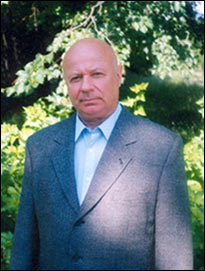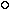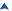Russian page– head of Department of Number Theory, Steklov Institute of Mathematics of Russian Academy of Sciences,

– professor of Department "Mathematical Analysis",
Faculty of Mechanics and Mathematics, M. V. Lomonosov Moscow State University (MSU)

Address: Department of Number Theory, Steklov Institute of Mathematics RAS
8, Gubkina str., 119991, Moscow, Russia

Tel.: (495) 938 37 32
Fax: (495) 135 05 55
Email: karatsuba@mi.ras.ru

Born 31.01.1937, GroznyScientific interestsEducation and Professional ActivitiesPrizes and AwardsList of Research WorksThe paper: G. I. Archipov, V. N. Chubarikov "On the mathematical works of professor A. A. Karatsuba"(Proc. Steklov Inst. Math., vol. 218, 1997).The paper: S. A. Gritsenko, E. A. Karatsuba, M. A. Korolev, I. S. Rezvyakova, D. I. Tolev, M. E. Changa, "Scientific Achievements of Anatolii Alexeevich Karatsuba" Proceedings of the Steklov Institute of Mathematics (Supplementary issues), 2013, 280, suppl. 2, S1–S22.The paper: E. A. Karatsuba, "Comments to My Works,Written by Myself" Proceedings of the Steklov Institute of Mathematics (Supplementary issues), 2013, 282, suppl. 1, S1–S23.Top
Scientific interests and works are in the field of analytic number theory and mathematical cybernetics:
1. Theory of trigonometric sums and trigonometric integrals:
• the Tarry problem
• multiple trigonometric sums
• estimating the Hardy function in the Waring problem
• the Artin problem on local representation of zero by a form
• the Hua Loo Keng problem on the index of convergence of the singular integral in the Tarry problem
• estimating the short Kloostermans sums
• a multidimensional analogue of Waring's problem
2. Theory of the Riemann zeta function:
• the Selberg problem
• zeros of linear combinations of L-series of Dirichlet
• distribution of zeros of the Riemann zeta function on the short intervals of the critical line
• the bound of zeros of the Riemann zeta function and the multidimensional Dirichlet divisor problem
• lower bounds for the maximum modulus of zeta function in small domains of the critical strip and in short intervals of the critical line
• the behavior of the argument of zeta function on the critical line
3. Theory of the Dirichlet characters:
• estimating sums of characters in finite fields
• estimating linear sums of characters in shifted prime numbers
• estimating sums of characters of polynomials with prime argument
• lower bounds for the sums of characters of polynomials
• sums of characters on additive sequences
• distribution of power residues and primitive roots in sparse sequences
4. Theory of finite automata:
• the problem of sharp estimate of the least length of the experiment determining the state of the automaton in the end of the experiment
5. Theory of fast computations:
• the first general method and algorithm for fast multiplication of multiplace numbers – the "divide and conquer" method which had served as the source of a new direction of investigations connected with fast computations
• the complexity of the computation of the functions.Top

#### Education and Professional ActivitiesTop

#### Prizes and AwardsTop